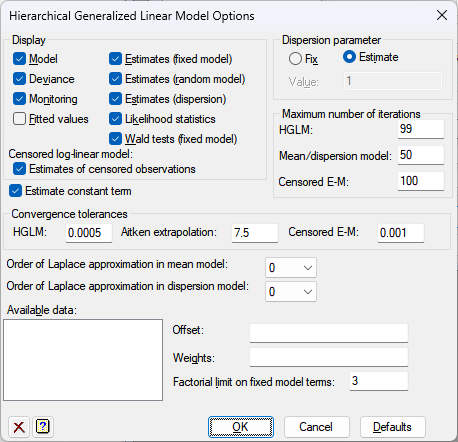1. Home
2. Hierarchical Generalized Linear Model Options

# Hierarchical Generalized Linear Model Options

Use this to set options and select the output to be generated by Hierarchical Generalized Linear Model analysis.## Display

This specifies which items of output are to be produced by the analysis.

 Model Details of the model that has been fitted Deviance Estimates of the deviances Monitoring Monitoring information at each iteration Fitted values Table with unit number, response variable, fitted values, residuals and leverages Estimates (fixed model) Estimates of the parameters in the fixed model Estimates (random model) Estimates of the parameters in the random model Estimates (dispersion) Estimates of the parameters in the dispersion models (if any) Likelihood statistics Likelihood statistics for assessing the models Wald tests (fixed model) Wald statistics for the terms in the fixed model Estimates of censored observations When censoring is selected for a Poisson-log link model, this prints the estimates of censored observations

## Dispersion parameter

Controls whether the dispersion parameter for the variance of the response is estimated from the residual mean square of the fitted model, or fixed at a given value. The dispersion parameter (fixed or estimated) is used when calculating standard errors and standardized residuals. In models with the binomial or Poisson distributions, the dispersion should be fixed at 1 unless a heterogeneity parameter is to be estimated.

## Estimate constant term

Specifies whether to include a constant in the HGLM fixed model. If the constant is not included, the intercept term will be zero.

## Maximum number of iterations

 GLM maximum number of iterations of the hierarchical generalized linear algorithm Mean dispersion model maximum number of iterations in the fitting of the mean and dispersion models Censored E-M maximum number of iterations in the fitting censored observations with the Tobit expectation-maximization algorithm

## Convergence tolerances

 HGLM maximum change in hierarchical generalized linear model parameters between iterations Aitken extrapolation maximum size of ratio of the original to the new estimates allowed in Aitken extrapolation Censored E-M maximum change in estimated censored observations with the Tobit expectation-maximization algorithm

## Order of Laplace approximation in mean model

This can be used to set the order of the Laplace approximation to use in the estimation of the mean model.

## Order of Laplace approximation in dispersion model

This can be used to set the order of the Laplace approximation involved in the estimation of the dispersion components of the model. This is appropriate for generalized linear mixed models with the binomial or Poisson distributions, where use of Laplace order 0 can lead to serious downwards bias. Caution must be used when setting an order of 2 as the analysis can take a long time to run.

## Offset

A fixed model in an HGLM can be modified to take account of a fixed contribution to the linear effects for each unit, supplied in a variate referred to as the offset.

## Weights

This lets you specify a variate of prior for the weights for the fixed model.

## Factorial limit on fixed model terms

For the fixed model you can control the factorial limit on model terms to be generated when you use model-formula operators like *. The default is to include all interactions, up to those involving nine variates or factors.

## Action buttons

 Run Save options and close dialog. Cancel Close dialog without saving the options. Defaults Reset the options to their default settings.

## Action IconsClear Clear all fields and list boxes.Help Open the Help topic for this dialog.